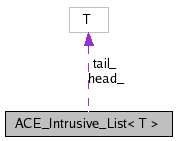# ACE_Intrusive_List< T > Class Template Reference

Implement an intrusive double linked list. More...

`#include <Intrusive_List.h>`

Collaboration diagram for ACE_Intrusive_List< T >:[legend]

List of all members.

## Public Member Functions

ACE_Intrusive_List (void)
~ACE_Intrusive_List (void)
Destructor.
bool is_empty (void) const
Returns true if the container is empty, otherwise returns false.
bool empty (void) const
void push_front (T *node)
Insert an element at the beginning of the list.
void push_back (T *node)
Insert an element at the end of the list.
T * pop_front (void)
Remove the element at the beginning of the list.
T * pop_back (void)
Remove the element at the end of the list.
Get the element at the head of the queue.
T * tail (void) const
Get the element at the tail of the queue.
void remove (T *node)
Remove a element from the list.
void swap (ACE_Intrusive_List< T > &rhs)
Swap two lists.
void unsafe_remove (T *node)
Remove a element from the list without checking.

## Private Member Functions

Disallow copying
ACE_Intrusive_List (const ACE_Intrusive_List< T > &)
ACE_Intrusive_List< T > & operator= (const ACE_Intrusive_List< T > &)

## Private Attributes

T * tail_
Tail of the list.

## Detailed Description

### template<class T> class ACE_Intrusive_List< T >

Implement an intrusive double linked list.

Intrusive lists assume that the elements they contain the pointers required to build the list. They are useful as light-weight containers and free-lists.

The template argument T must implement the following methods:

• T* T::next () const;
• void T::next (T *);
• T* T::prev () const;
• void T::prev (T* );

A simple way to satisfy the Intrusive_List requirements would be to implement a helper class:

class My_Object : public ACE_Intrusive_List_Node<My_Object> {
....
};

typedef ACE_Intrusive_List<My_Object> My_Object_List;

However, ACE is supported on platforms that would surely get confused using such templates.

Todo:
The ACE_Message_Queue is an example of an intrusive list (or queue) but it is not implemented in terms of this class.

## Constructor & Destructor Documentation

template<class T >
 ACE_Intrusive_List< T >::ACE_Intrusive_List ( void ) ` [inline]`

Constructor. Use user specified allocation strategy if specified.

template<class T >
 ACE_Intrusive_List< T >::~ACE_Intrusive_List ( void ) ` [inline]`

Destructor.

template<class T>
 ACE_Intrusive_List< T >::ACE_Intrusive_List ( const ACE_Intrusive_List< T > & ) ` [private]`

## Member Function Documentation

template<class T >
 bool ACE_Intrusive_List< T >::empty ( void ) const` [inline]`

Returns true if the container is empty, otherwise returns false.

Deprecated:

template<class T >
 T * ACE_Intrusive_List< T >::head ( void ) const` [inline]`

Get the element at the head of the queue.

template<class T >
 bool ACE_Intrusive_List< T >::is_empty ( void ) const` [inline]`

Returns true if the container is empty, otherwise returns false.

template<class T>
 ACE_Intrusive_List& ACE_Intrusive_List< T >::operator= ( const ACE_Intrusive_List< T > & ) ` [private]`

template<class T >
 T * ACE_Intrusive_List< T >::pop_back ( void ) ` [inline]`

Remove the element at the end of the list.

template<class T >
 T * ACE_Intrusive_List< T >::pop_front ( void ) ` [inline]`

Remove the element at the beginning of the list.

template<class T>
 void ACE_Intrusive_List< T >::push_back ( T * node ) ` [inline]`

Insert an element at the end of the list.

template<class T>
 void ACE_Intrusive_List< T >::push_front ( T * node ) ` [inline]`

Insert an element at the beginning of the list.

template<class T>
 void ACE_Intrusive_List< T >::remove ( T * node ) ` [inline]`

Remove a element from the list.

Verify that the element is still in the list before removing it.

template<class T>
 void ACE_Intrusive_List< T >::swap ( ACE_Intrusive_List< T > & rhs ) ` [inline]`

Swap two lists.

template<class T >
 T * ACE_Intrusive_List< T >::tail ( void ) const` [inline]`

Get the element at the tail of the queue.

template<class T>
 void ACE_Intrusive_List< T >::unsafe_remove ( T * node ) ` [inline]`

Remove a element from the list without checking.

No attempts are performed to check if T* really belongs to the list. The effects of removing an invalid element are unspecified

## Member Data Documentation

template<class T>
 T* ACE_Intrusive_List< T >::head_` [private]`

 T* ACE_Intrusive_List< T >::tail_` [private]`
Generated on Tue Apr 14 11:11:39 2009 for ACE by1.5.8